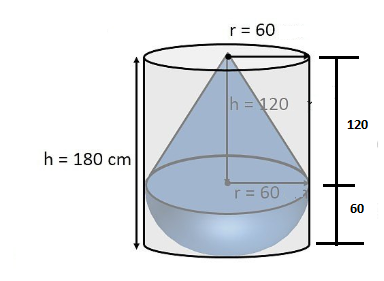Newbie

# A solid consisting of a right circular cone of height 120 cm and radius 60 cm standing on a hemisphere of radius 60 cm is placed upright in a right circular cylinder full of water such that it touches the bottom. Find the volume of water left in the cylinder, if the radius of the cylinder is 60 cm and its height is 180 cm.Q.7

• 0

How  i solve the ncert class 10 questions of  surface areas and volumes .Find the solution of exercise 13.2 of question number 7 . Please help me to solve the simplest method of this question.A solid consisting of a right circular cone of height 120 cm and radius 60 cm standing on a hemisphere of radius 60 cm is placed upright in a right circular cylinder full of water such that it touches the bottom. Find the volume of water left in the cylinder, if the radius of the cylinder is 60 cm and its height is 180 cm.

Share

1. Solution:Here, the volume of water left will be = Volume of cylinder – Volume of solid

Given,

Radius of cone = 60 cm,

Height of cone = 120 cm

Radius of cylinder = 60 cm

Height of cylinder = 180 cm

Radius of hemisphere = 60 cm

Now,

Total volume of solid = Volume of Cone + Volume of hemisphere

Volume of cone = π×122×103cm3 = 144×103π cm3

So, Total volume of solid = 144×103π cm-(⅔)×π×10cm3

Volume of hemisphere = (⅔)×π×10cm3

Volume of cylinder = π×602×180 = 648000 = 648×103 π cm3

Now, volume of water left will be = Volume of cylinder – Volume of solid

= (648-288) × 103×π = 1.131 m3

• 0